### IMO Shortlist 2002 problem C1

Kvaliteta:
Avg: 4,0
Težina:
Avg: 6,0
Let$n$ be a positive integer. Each point$(x,y)$ in the plane, where$x$ and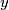$y$ are non-negative integers with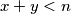$x+y, is coloured red or blue, subject to the following condition: if a point$(x,y)$ is red, then so are all points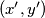$(x',y')$ with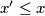$x'\leq x$ and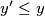$y'\leq y$. Let$A$ be the number of ways to choose$n$ blue points with distinct$x$-coordinates, and let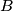$B$ be the number of ways to choose$n$ blue points with distinct$y$-coordinates. Prove that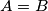$A=B$.
Izvor: Međunarodna matematička olimpijada, shortlist 2002